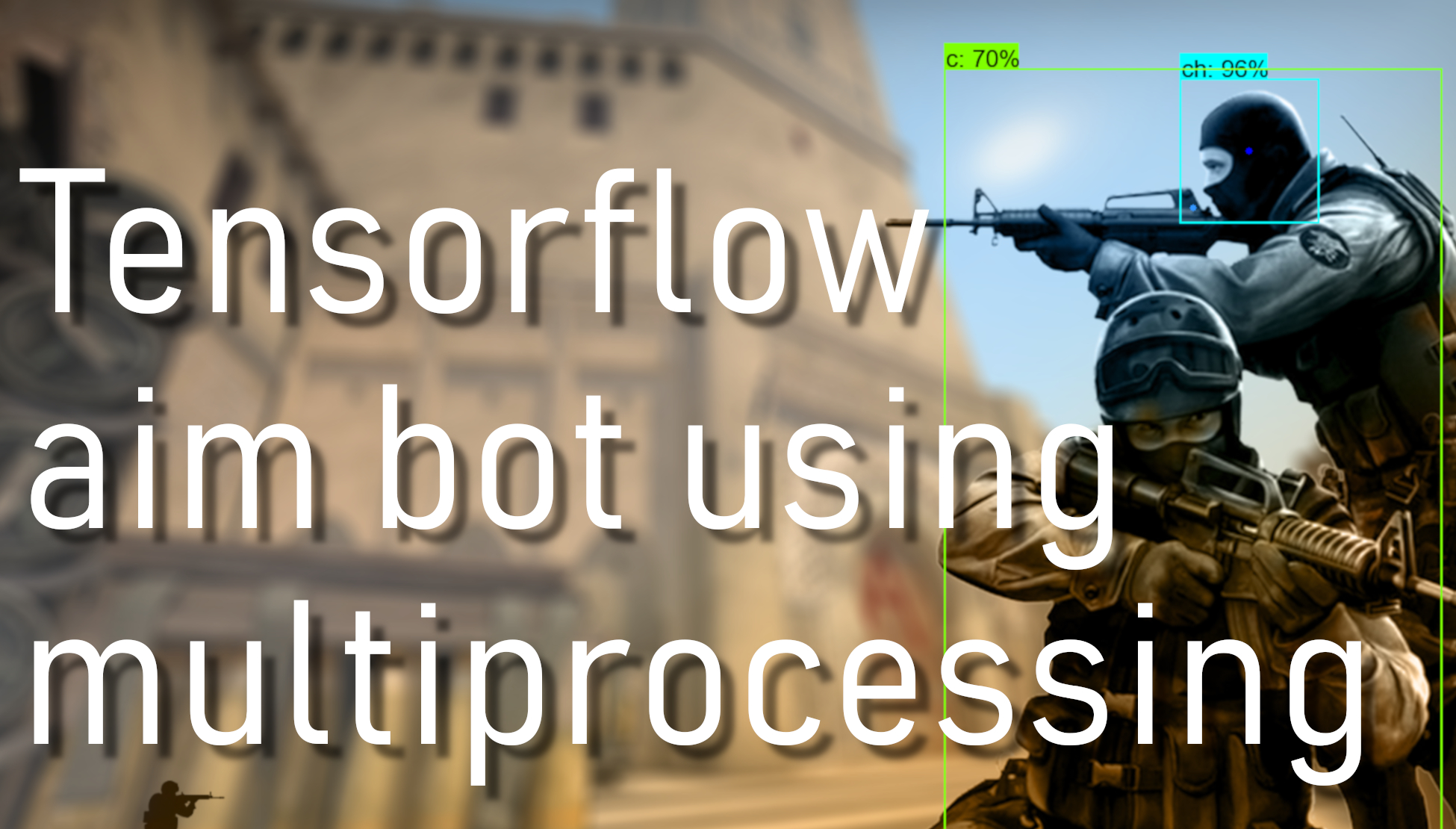# TensorFlow Object Detection aimbot with multiprocessing

## In this tutorial, I will merge multiprocessing code with TensorFlow object detection code to maximize FPS performance

Welcome everyone to part 10 of our TensorFlow object detection API tutorial series. First, you can download the code on my GitHub page. This will be the final tutorial of this CSGO aimbot video series because right now, I spent too much time on this tutorial. Nevertheless, I managed to get the best performance I can for now. For further improvements, I would need to investigate time to another detection method - that I will do in the future.

Before continuing on this tutorial, I should mention that I updated the 9th tutorial code before merging it with the CSGO TensorFlow detection code. Compared with multiprocessing queues, performance is the same (33FPS), but I wanted to test different data methods to share data between processes. So I updated the 9th tutorial, added one more file in which we are grabbing screen by using multiprocessing pipes. Adding to multiprocessing pipes, they use communication one to one, and queues may be used as "many to many".

Continuing on this tutorial, I am not going to code explanation in this text tutorial part. Instead, I divided the whole code into 3 parts: grabbing the screen, making TensorFlow detection, and showing the screen. Then, all these 3 parts were moved to multiprocessing processes.

Here is the final code:

``````# # Imports
import multiprocessing
from multiprocessing import Pipe
import time
import cv2
import mss
import numpy as np
import os
import sys
os.environ['CUDA_VISIBLE_DEVICES'] = '0'
import tensorflow as tf
from distutils.version import StrictVersion
from collections import defaultdict
from io import StringIO
import pyautogui

# title of our window
title = "FPS benchmark"
# set start time to current time
start_time = time.time()
# displays the frame rate every 2 second
display_time = 2
# Set primarry FPS to 0
fps = 0
# Load mss library as sct
sct = mss.mss()
# Set monitor size to capture to MSS
width = 800
height = 640

monitor = {"top": 80, "left": 0, "width": width, "height": height}

# ## Env setup
from object_detection.utils import ops as utils_ops
from object_detection.utils import label_map_util
from object_detection.utils import visualization_utils as vis_util

# # Model preparation
PATH_TO_FROZEN_GRAPH = 'CSGO_frozen_inference_graph.pb'
# List of the strings that is used to add correct label for each box.
PATH_TO_LABELS = 'CSGO_labelmap.pbtxt'
NUM_CLASSES = 4

# ## Load a (frozen) Tensorflow model into memory.
categories = label_map_util.convert_label_map_to_categories(label_map, max_num_classes=NUM_CLASSES, use_display_name=True)
category_index = label_map_util.create_category_index(categories)

detection_graph = tf.Graph()
with detection_graph.as_default():
od_graph_def = tf.GraphDef()
with tf.gfile.GFile(PATH_TO_FROZEN_GRAPH, 'rb') as fid:
od_graph_def.ParseFromString(serialized_graph)
tf.import_graph_def(od_graph_def, name='')

def Shoot(mid_x, mid_y):
x = int(mid_x*width)
#y = int(mid_y*height)
y = int(mid_y*height+height/9)
pyautogui.moveTo(x,y)
pyautogui.click()

def grab_screen(p_input):
while True:
#Grab screen image
img = np.array(sct.grab(monitor))
img = cv2.cvtColor(img, cv2.COLOR_BGR2RGB)
img = cv2.cvtColor(img, cv2.COLOR_BGR2RGB)

# Put image from pipe
p_input.send(img)

def TensorflowDetection(p_output, p_input2):
# Detection
with detection_graph.as_default():
with tf.Session(graph=detection_graph) as sess:
while True:
# Get image from pipe
image_np = p_output.recv()
# Expand dimensions since the model expects images to have shape: [1, None, None, 3]
image_np_expanded = np.expand_dims(image_np, axis=0)
# Actual detection.
image_tensor = detection_graph.get_tensor_by_name('image_tensor:0')
boxes = detection_graph.get_tensor_by_name('detection_boxes:0')
scores = detection_graph.get_tensor_by_name('detection_scores:0')
classes = detection_graph.get_tensor_by_name('detection_classes:0')
num_detections = detection_graph.get_tensor_by_name('num_detections:0')
# Visualization of the results of a detection.
(boxes, scores, classes, num_detections) = sess.run(
[boxes, scores, classes, num_detections],
feed_dict={image_tensor: image_np_expanded})
vis_util.visualize_boxes_and_labels_on_image_array(
image_np,
np.squeeze(boxes),
np.squeeze(classes).astype(np.int32),
np.squeeze(scores),
category_index,
use_normalized_coordinates=True,
line_thickness=2)

# Send detection image to pipe2
p_input2.send(image_np)

array_ch = []
array_c = []
array_th = []
array_t = []
for i,b in enumerate(boxes):
if classes[i] == 2: # ch
if scores[i] >= 0.5:
mid_x = (boxes[i]+boxes[i])/2
mid_y = (boxes[i]+boxes[i])/2
array_ch.append([mid_x, mid_y])
cv2.circle(image_np,(int(mid_x*width),int(mid_y*height)), 3, (0,0,255), -1)
if classes[i] == 1: # c
if scores[i] >= 0.5:
mid_x = (boxes[i]+boxes[i])/2
mid_y = boxes[i] + (boxes[i]-boxes[i])/6
array_c.append([mid_x, mid_y])
cv2.circle(image_np,(int(mid_x*width),int(mid_y*height)), 3, (50,150,255), -1)
if classes[i] == 4: # th
if scores[i] >= 0.5:
mid_x = (boxes[i]+boxes[i])/2
mid_y = (boxes[i]+boxes[i])/2
array_th.append([mid_x, mid_y])
cv2.circle(image_np,(int(mid_x*width),int(mid_y*height)), 3, (0,0,255), -1)
if classes[i] == 3: # t
if scores[i] >= 0.5:
mid_x = (boxes[i]+boxes[i])/2
mid_y = boxes[i] + (boxes[i]-boxes[i])/6
array_t.append([mid_x, mid_y])
cv2.circle(image_np,(int(mid_x*width),int(mid_y*height)), 3, (50,150,255), -1)

team = "c" # shooting target
if team == "c":
if len(array_ch) > 0:
Shoot(array_ch, array_ch)
if len(array_ch) == 0 and len(array_c) > 0:
Shoot(array_c, array_c)
if team == "t":
if len(array_th) > 0:
Shoot(array_th, array_th)
if len(array_th) == 0 and len(array_t) > 0:
Shoot(array_t, array_t)

def Show_image(p_output2):
global start_time, fps
while True:
image_np = p_output2.recv()
# Show image with detection
cv2.imshow(title, image_np)
# Bellow we calculate our FPS
fps+=1
TIME = time.time() - start_time
if (TIME) >= display_time :
print("FPS: ", fps / (TIME))
fps = 0
start_time = time.time()
# Press "q" to quit
if cv2.waitKey(25) & 0xFF == ord("q"):
cv2.destroyAllWindows()
break

if __name__=="__main__":
# Pipes
p_output, p_input = Pipe()
p_output2, p_input2 = Pipe()

# creating new processes
p1 = multiprocessing.Process(target=grab_screen, args=(p_input,))
p2 = multiprocessing.Process(target=TensorflowDetection, args=(p_output,p_input2,))
p3 = multiprocessing.Process(target=Show_image, args=(p_output2,))

# starting our processes
p1.start()
p2.start()
p3.start()``````

As a final result, I was quite happy that we could achieve more than 20 FPS. But when TensorFlow receives images where we detect enemies, here comes the bottleneck. FPS drops to 4-5 frames per second, and it becomes impossible to play this game for our bot. So in the future, I may come back to this project when I find methods to detect our enemies faster. There is a way to use the YOLO object detection model, it's quite fast and accurate, but it's harder to implement (for now).

Anyway, I think I spent a lot of time working on this project. Right now, I will move to another more beneficial project. In nearest future, I am planning to make tutorials on how to crack CAPTCHA's, how to use SELENIUM to make web surfing bots or AI forex trading bot.